Courses

# Electrostatic, JEE Main Notes JEE Notes | EduRev

## JEE : Electrostatic, JEE Main Notes JEE Notes | EduRev

The document Electrostatic, JEE Main Notes JEE Notes | EduRev is a part of the JEE Course Physics For JEE.
All you need of JEE at this link: JEE

What is Electrostatics?

It is a branch of physics that deals with the phenomena and properties of stationary or slow-moving electric charges with no acceleration.

• A pith ball electroscope is used to detect a charge and to know the nature of the charge.
• Gold leaf electroscope which was invented by Bennet detects a charge and the nature of the charge and determines the quantity of the charge.

Conductors, Insulators, and Semiconductors

• A body in which electric charge can easily flow through is called a conductor (e.g. metals).
• A body in which electric charge cannot flow is called an insulator or dielectric. (e.g. glass, wood, rubber, plastic, etc)
• Substances that are intermediate between conductors and insulators are called semiconductors. (e.g. silicon, germanium, etc)

What is an Electric charge?

The property associated with matter due to which it produces and experiences electric and magnetic fields.

Properties of charge

• Transferable
• Always associated with mass
• Conserved
• Quantized

Methods of charging of a body

• Conduction
• Induction
• Friction
1. Charging a body by conduction: The process of charging by bringing a charged body in contact with a neutral body is called charging by conduction. We observe that both bodies involved in this method of charging by conduction acquire the same type of charge once the process is complete.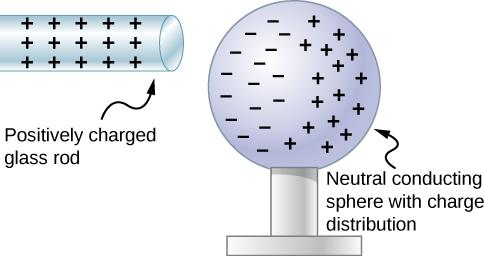2. Charging a body by induction: When a charged object, 'A' is placed near an uncharged object B, object A induces an unlike charge on the near side of B and a like charge on its far side. In this case, the charges inside B are only polarised and no transfer of charges takes place. In this method, we observe that the induced charge is always equal to the inducing charge for a conductor.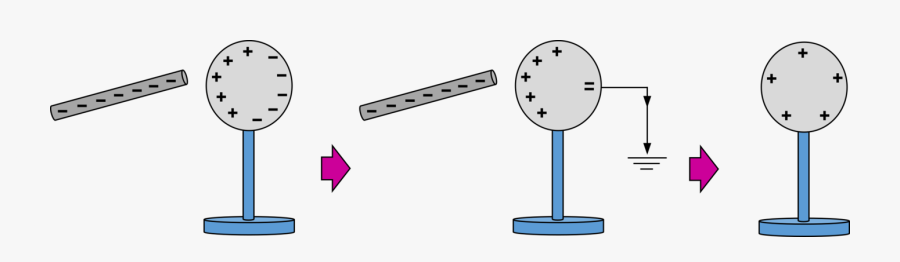3. Charging a body by friction: When two uncharged or neutral objects made of different materials, say a glass rod and a silk cloth, are rubbed against each other, both objects get electrified due to a transfer of charges. When rubbed, the glass rod loses a few electrons to the silk cloth. This deficit of electrons on the glass rod makes it positively charged and the excess of electrons makes the silk cloth negatively charged. In this method, there is a transfer of electrons from one body to another. The material that gains electrons is negatively charged and the one that loses electrons is positively charged.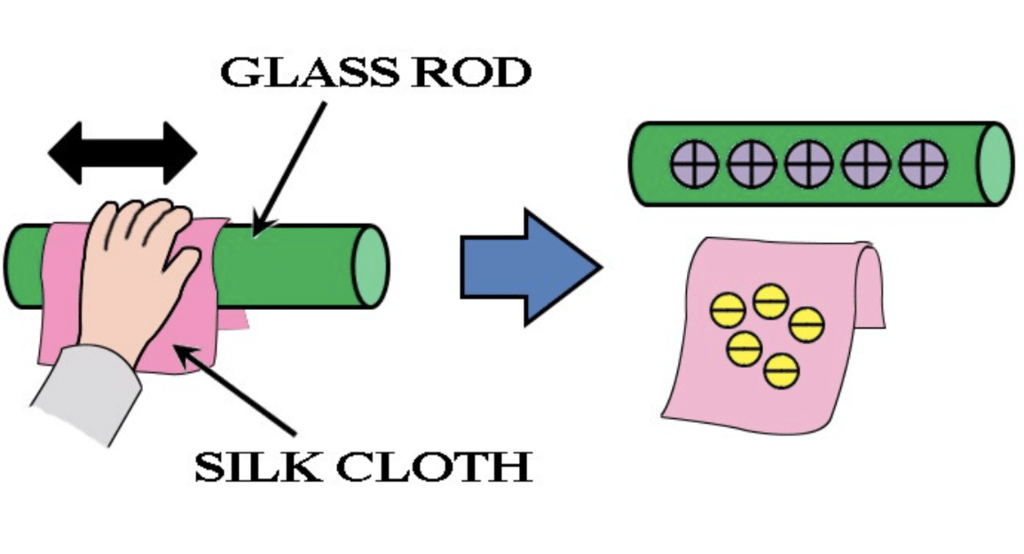What is Coulomb’s Law?

It states that the electrostatic force of attraction or repulsion between two charged bodies is directly proportional to the product of their charges and varies inversely as the square of the distance between the two bodies.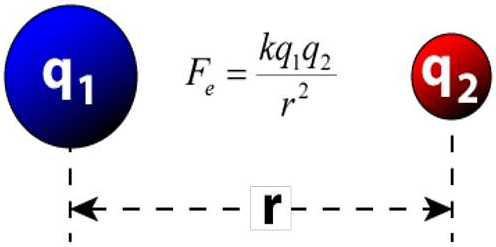Here,

K = 1/(4πε0) = 9×109 Nm2C-2 (in free space)

Relative Permittivity (εr)

The relative permittivity (εr) of a medium is defined as the ratio between its permittivity of the medium (ε) and the permittivity (ε0) of the free space.
i.e. εr = ε/ε0

Coulomb force in vector form

The force on charge q1 due to q2 is,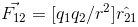If q1q2>0, R.H.S is positive but if q1q2<0, a negative sign from q1 or q2 will change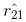to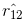.  The relation will again be true, since, in that case, have the same directions.

Superposition Principle

The total force on a given charge is the vector sum of all the individual forces exerted by each of the other charges.

Unit of Charge

• C.G.S., q = ±1 stat-coulomb
• S.I., q = ±1 Coulomb

Relation between coulomb and stat-coulomb

• 1 coulomb = 3×109 stat-coulomb
• 1 coulomb =(1/10) ab-coulomb (e.m.u of charge)

What is the Dielectric constant?

The dielectric constant (εr) of a medium can be defined as the ratio of the force between two charges separated by some distance apart in free space to the force between the same two charges separated by the same distance apart in that medium.
i.e. ε= ε/ε0 = F1/F2

Here, F1 and F2 are the magnitudes of the force between them in free space and in a medium respectively.

Charges

• Line charge density, λ = q/L
• Surface charge density, σ = q/A
• Volume charge density, ρ = q/V

What is an Electric field (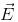)?

The strength of an electric field is measured by the force experienced by a unit positive charge placed at that point. The direction of the field is given by the direction of motion of a unit positive charge if it were free to move.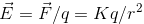For positive source charge, the electric field is radially outward, whereas, for negative source charge, the electric field is radially inward.

Unit of Electric field

E = [Newton/Coulomb] or [Joule/(Coulomb) (meter)]

What are Electric lines of force?

An electric line of force is defined as the path, straight or curved, along which a unit positive charge is urged to move when free to do so in an electric field. The direction of motion of the unit positive charge gives the direction of the line of force.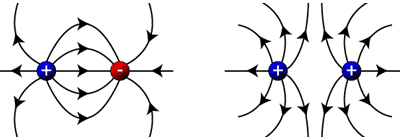Properties

• The lines of force are directed away from a positively charged conductor and are directed towards a negatively charged conductor.
• A line of force starts from a positive charge and ends on a negative charge. This signifies line of force starts from higher potential and ends on lower potential.

What is Electric Flux?

Electric flux for a surface placed in an electric field is the sum of dot product of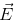and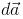for all the elementary areas constituting the surface.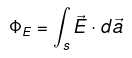What is Gauss Theorem?

It states that, for any distribution of charges, the total electric flux linked with a closed surface is 1/ε0 times the total charge with in the surface.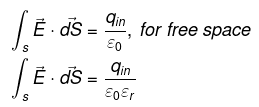What is Electric Field Intensity?

The space around an electric charge in which its influence can be felt is known as the electric field. The electric field intensity at a point is the force experienced by a unit positive charge placed at that point.

Electric Field Intensity (E) = q/[4πεd2]NC-1

• Electric field intensity due to a point charge:

E = (1/4πε0) (q/r2)

• Electric field Intensity due to a linear distribution of charge: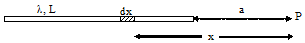(a) At point on its axis, E = (λ/4πε0) [1/a – 1/a+L]
Here, λ is the linear charge density.
(b) At a point on the line perpendicular to one end,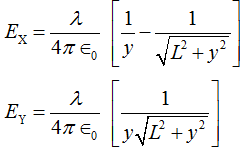Here, λ is the line charge density.

• Electric field (E) of an infinite rod at a distance (r) from the line having linear charge density (λ):

E = λ/2πε0r

The direction of electric field E is radially outward for a line of positive charge.

• Electric field due to ring of uniform charge distribution: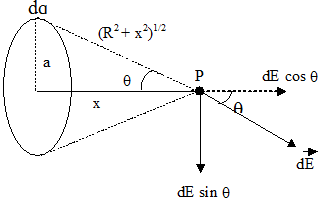At a point on its axis, E = (1/4πε0) [qx/(a+ x2)3/2]
• Electric field due to uniformly charged disc: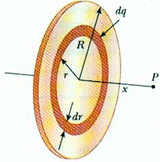At a point on its axis, E = (1/4πε0)(2πσ)[1 - x/(a2+R2)1/2]
Here, σ is the surface charge.

• Electric field due to thin spherical shell: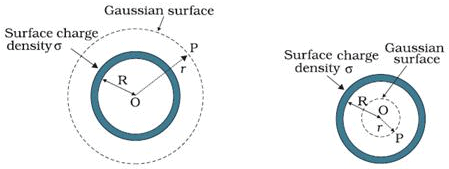1. Outside Point: Eout = (1/4πε0) (q/r2)
2. Inside Point: Ein = 0

• The electric field of a spherically symmetric distribution of charge of Radius R: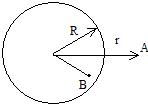1. Point at outside (r > R): E = (1/4πε0) (q/r2)
2. Point at inside (r < R): E = (1/4πε0) (qr/R3)
Here, q is the total charge.

• The electric field of a non-conducting solid sphere having uniform volume distribution of charge: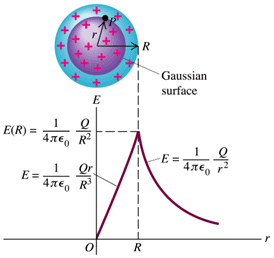1. Outside Point: Eout = (1/4πε0) (Q/r2)
2. Inside Point: Ein = (1/4πε0) (Qr/R3)
3. On the Surface: Esurface = (1/4πε0) (Q/R2)

Here, Q is the total charge

• The electric field of a cylindrical conductor of infinite length having line charge λ: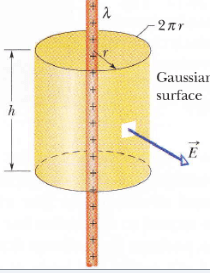1. Outside the cylinder: E = λ/(2πε0r)
2. Inside the cylinder: E = 0

• The electric field of a non-conducting cylinder having uniform volume density of charge:
1. Outside the cylinder: E = λ/2πε0r
2. Inside the cylinder: E = ρr/2ε0

• The electric field of an infinite plane sheet of charge surface charge (σ):

E = σ/2ε0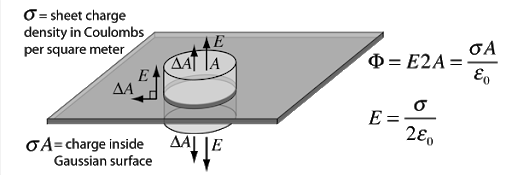• Electric field due to two oppositely infinite charged sheets: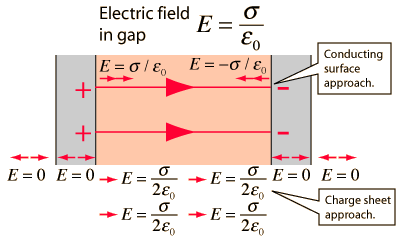1. Electric field at points outside the charged sheets: EP = ER = 0
2. Electric field at a point in between the charged sheets: EQ = σ/ε0

• Electric field due to an infinite non-conducting flat sheet having charge σ:

E = σ/2ε0
This signifies, the electric field near a charged sheet is independent of the distance of the point from the sheet and depends only upon its charge density and is directed normally to the sheet.

• Electric field due to an infinite flat conductor carrying charge:

E= σ/ε0

• Electric pressure (Pelec) on a charged conductor:

Pelec = (1/2 ε0) σ2

What is Electric Dipole?

An electric dipole is defined as a couple of opposite charges q and –q separated by a distance d.

• By default, the direction of electric dipoles in space is always from negative charge -q to positive charge q.
• The midpoint q and –q is called the centre of the dipole.
• The simplest example of an electric dipole is a pair of electric charges of two opposite signs and equal magnitude separated by distance.

What is Dipole Moment?

Dipole moment (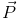) of an electric dipole is defined as the product of the magnitude of one of the charges and the vector distance from negative to positive charge.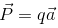Unit of Dipole Moment:

• S.I.: p = coulomb-meter
• C.G.S.: p = stat-coulomb-cm

Electric field due to an electric dipole:

(a) At any point on the axial line: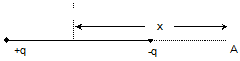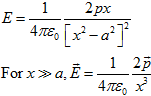(b) At a point on the equatorial line (perpendicular bisector):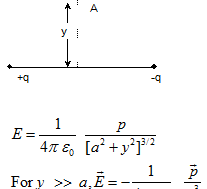(c) At any point: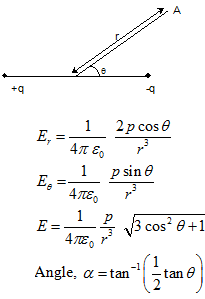Torque (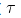) acting on an electric dipole in a uniform electric field (E):= pE sinθ

Here, p is the dipole moment and θ is the angle between the direction of dipole moment and electric field E.

What is Electro-Static Potential?

Electric potential, at any point, is defined as the negative line integral of the electric field from infinity to that point along any path.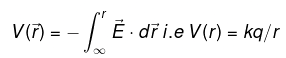The potential difference, between any two points, in an electric field, is defined as the work done in taking a unit positive charge from one point to the other against the electric field.
WAB = q [VA-VB]
So, V = [VA-VB] = W/q

Units & Dimension

• S.I.: V= volt
• C.G.S.: V = stat-volt
• Dimension: [V] = [ML2T-3A-1]
• Relation between volt and stat-volt: 1 volt = (1/300) stat-volt
• Relation between electric field (E) and electric potential (V): E = -dV/dx = --dV/dr

Potential due to a point charge

V = (1/4π ε0) (q/r)

Potential at a point due to several charges

V = (1/4π ε0) [q1/r1 + q2/r2 + q3/r3] = V1+V2+ V2+….

Potential due to charged spherical shell

1. Outside, Vout = (1/4π ε0) (q/r)
2. Inside, Vin = - (1/4π ε0) (q/R)
3. On the surface, Vsurface = (1/4π ε0) (q/R)

Potential due to a uniformly charged non-conducting sphere

1. Outside, Vout = (1/4π ε0) (q/r)
2. Inside, Vin = (1/4π ε0) [q(3R2-r2)/2R3]
3. On the surface, Vsurface = (1/4π ε0) (q/R)
4. In center, Vcenter = (3/2) [(1/4π ε0) (q/R)] = 3/2 [Vsurface]

Common potential (two spheres joined by thin wire):

Common potential, V = (1/4π ε0) [(Q1+Q2)/(r1+r2)]
q= r1(Q1+Q2)/(r1+r2) = r1Q/ r1+r2
q2 =  r2Q/ r1+r2
q1/q2 = r1/r2 or σ1/ σ2 = r1/r2

Potential at any point due to an electric dipole:

V (r,θ) = qa cosθ/(4πε0r2) = p cosθ/(4πε0r2)
1. Point lying on the axial line: V = p/4πε0r2
2. Point situated on equatorial lines: V = 0

If n drops coalesce to form one drop, then,

• R = n1/3r
• Q = nq
• V = n2/3Vsmall
• σ = n1/3 σsmall
• E = n1/3 Esmall

Electric potential energy U or work done of the system W having charge q1 and q2

W = U = 1/(4πε0) (q1q2/r12) = q1V1

Electric potential energy U or work done of the system W of a three-particle system having charge q1, q2 and q3

W = U = (1/4πε0) (q1q2/r12 + q1q3/r13 + q2q3/r23)

The electric potential energy of an electric dipole in an electric field

Potential energy of an electric dipole, in an electrostatic field, is defined as the work done in rotating the dipole from zero energy position to the desired position in the electric field.

•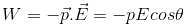If θ = 90º, then W = 0
• If θ = 0º, then W = -pE
• If θ = 180º, then W = pE

The kinetic energy of a charged particle moving through a potential difference:

K. E = 1/2 mv2 = eV

Capacitance

• Conductors

Conductors are those substances through which electric charge flow easily.

• Insulators:

Insulators (also called dielectrics) are those substances through which electric charge cannot flow easily.

• Capacity:

The capacity of a conductor is defined as the ratio between the charge of the conductor to its potential.
C = Q/V

Units & Dimension

• S.I.: C = farad (coulomb/volt)
• C.G.S.: C = stat-farad (stat-coulomb/stat-volt)
• Dimension: [M-1L-2T4A2]

The capacity of an isolated spherical conductor:

C = 4πε0r

• Capacitor:

A capacitor or a condenser is an arrangement that provides a larger capacity in a smaller space.

• The capacity of a parallel plate capacitor:

Cair = ε0A/d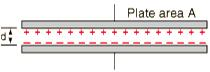Cmed = Kε0A/d
Here, A is the common area of the two plates and d is the distance between the plates.

• Effect of dielectric on the capacitance of a capacitor:

C = ε0A/[d-t+(t/K)]
Here d is the separation between the plates, t is the thickness of the dielectric slab, A is the area and K is the dielectric constant of the material of the slab.
If the space is completely filled with dielectric medium (t=d), then, C = ε0KA/ d

• The capacitance of a sphere:
1. Cair = 4πε0R
2. Cmed = K (4πε0R)
• The capacity of a spherical condenser:
1. When outer sphere is earthed:
Cair = 4πε0 [ab/(b-a)]
Cmed = 4πε[Kab/(b-a)]
2. When the inner sphere is earthed:
C1= 4πε0 [ab/(b-a)]
C2 = 4πε0b
Net Capacity, C '=4πε0[b2/b-a]

Increase in capacity, ΔC = 4π ε0b
It signifies, by connecting the inner sphere to earth and charging the outer one we get an additional capacity equal to the capacity of the outer sphere.

• The capacity of a cylindrical condenser:
1. Cair = λl / [(λ/2π ε0) (loge b/a)] = [2πε0l /(loge b/a) ]
2. Cmed = [2πKε0l /(loge b/a) ]
• The potential energy of a charged capacitor (Energy stored in a capacitor)

W = ½ QV = ½ Q2/C = ½ CV2

• The energy density of a capacitor:

U = ½ ε0E2 = ½ (σ2/ ε0)

This signifies the energy density of a capacitor is independent of the area of plates and the distance between them so, the value of E does not change.

Grouping of Capacitors

1. Capacitors in parallel:

• C = C1+C2+C3+…..+Cn
The resultant capacity of a number of capacitors, connected in parallel, is equal to the sum of their individual capacities.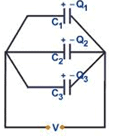• V1= V2= V3 = V
• q1 =C1V, q2 = C2V,  q3 = C3V
• Energy Stored, U = U1+U2+U3

2. Capacitors in Series:

• 1/C = 1/C1 + 1/ C2 +……+ 1/Cn
• The reciprocal of the resultant capacity of a number of capacitors, connected in series, is equal to the sum of the reciprocals of their individual capacities.
•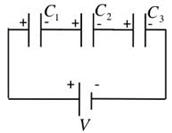• q= q2 = q3 = q
• V1= q/C1, V2= q/C2, V3= q/C3
• Energy Stored, U = U1+U2+U3

Energy stored in a group of capacitors:

1. Energy stored in a series combination of capacitors:
W = ½ (q2/C1) + ½ (q2/C2) + ½ (q2/C3) = W1+W2+W3
Thus, net energy stored in the combination is equal to the sum of the energies stored in the component capacitors.
2. Energy stored in a parallel combination of capacitors:
W = ½ C1V+½ C2V2 + ½ C3V2 = W1+W2+W3
The net energy stored in the combination is equal to the sum of energies stored in the component capacitors.

Force of attraction between plates of a charged capacitor:

(a) F = ½ ε0E2A
(b) F = σ2A/2ε0
(c) F=Q2/2ε0A

Force on a dielectric in a capacitor:

F = (Q2/2C2) (dC/dx) = ½ V(dC/dx)

Common potential when two capacitors are connected

V = [C1V1+ C2V2] / [C1+C2] = [Q1+Q2]/ [C1+C2]

Charge transfer when two capacitors are connected

ΔQ = [C1C2/C1+C2] [V1-V2]

Energy loss when two capacitors are connected

ΔU = ½ [C1C2/C1+C2] [V1-V2] 2

Charging of a capacitor

1. Q = Q0(1-e-t/RC)
2. V = V0(1-e-t/RC)
3. I = I0(1-e-t/RC)
4. I0 = V0/R

Discharging of a capacitor

1. Q = Q0(e-t/RC)
2. V = V0(e-t/RC)
3. I = I0(e-t/RC)

Time constant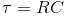Offer running on EduRev: Apply code STAYHOME200 to get INR 200 off on our premium plan EduRev Infinity!

## Physics For JEE

187 videos|516 docs|263 tests

,

,

,

,

,

,

,

,

,

,

,

,

,

,

,

,

,

,

,

,

,

,

,

,

;